## Elliptic Curve

Informally, an elliptic curve is a type of Cubic Curve whose solutions are confined to a region of space which is topologically equivalent to a Torus. Formally, an elliptic curve over a Field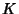is a nonsingular Cubic Curve in two variables,, with a-rational point (which may be a point at infinity). The Fieldis usually taken to be the Complex Numbers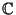, Reals, Rationals, algebraic extensions of, p-adic Number, or a Finite Field.

By an appropriate change of variables, a general elliptic curve over a Field of Characteristic(1)

where,, ..., are elements of, can be written in the form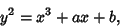(2)

where the right side of (2) has no repeated factors. Ifhas Characteristic three, then the best that can be done is to transform the curve into(3)

(theterm cannot be eliminated). Ifhas Characteristic two, then the situation is even worse. A general form into which an elliptic curve over anycan be transformed is called the Weierstraß Form, and is given by(4)

where,,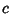,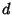, and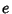are elements of. Luckily,,, andall have Characteristic zero.

Whereas Conic Sections can be parameterized by the rational functions, elliptic curves cannot. The simplest parameterization functions are Elliptic Functions. Abelian Varieties can be viewed as generalizations of elliptic curves.If the underlying Field of an elliptic curve is algebraically closed, then a straight line cuts an elliptic curve at three points (counting multiple roots at points of tangency). If two are known, it is possible to compute the third. If two of the intersection points are-Rational, then so is the third. Let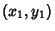and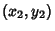be two points on an elliptic curve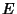with Discriminant(5)

satisfying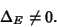(6)

A related quantity known as the j-Invariant ofis defined as(7)

Now define(8)

Then the coordinates of the third point are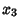(9)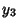(10)

For elliptic curves over, Mordell proved that there are a finite number of integral solutions. The Mordell-Weil Theorem says that the Group of Rational Points of an elliptic curve overis finitely generated. Let the Roots ofbe,, and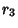. The discriminant is then(11)

The amazing Taniyama-Shimura Conjecture states that all rational elliptic curves are also modular. This fact is far from obvious, and despite the fact that the conjecture was proposed in 1955, it was not even partially proved until 1995. Even so, Wiles' proof of the semistable case surprised most mathematicians, who had believed the conjecture unassailable. As a side benefit, Wiles' proof of the Taniyama-Shimura Conjecture also laid to rest the famous and thorny problem which had baffled mathematicians for hundreds of years, Fermat's Last Theorem.

Curves with small Conductors are listed in Swinnerton-Dyer (1975) and Cremona (1997). Methods for computing integral points (points with integral coordinates) are given in Gebel et al. and Stroeker and Tzanakis (1994).

See also Elliptic Curve Group Law, Fermat's Last Theorem, Frey Curve, j-Invariant, Minimal Discriminant, Mordell-Weil Theorem, Ochoa Curve, Ribet's Theorem, Siegel's Theorem, Swinnerton-Dyer Conjecture, Taniyama-Shimura Conjecture, Weierstraß Form

References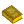Elliptic Curves

Atkin, A. O. L. and Morain, F. Elliptic Curves and Primality Proving.'' Math. Comput. 61, 29-68, 1993.

Cassels, J. W. S. Lectures on Elliptic Curves. New York: Cambridge University Press, 1991.

Cremona, J. E. Algorithms for Modular Elliptic Curves, 2nd ed. Cambridge, England: Cambridge University Press, 1997.

Cremona, J. E. Elliptic Curve Data.'' ftp://euclid.ex.ac.uk/pub/cremona/data/.

Du Val, P. Elliptic Functions and Elliptic Curves. Cambridge: Cambridge University Press, 1973.

Gebel, J.; Pethö, A.; and Zimmer, H. G. Computing Integral Points on Elliptic Curves.'' Acta Arith. 68, 171-192, 1994.

Ireland, K. and Rosen, M. Elliptic Curves.'' Ch. 18 in A Classical Introduction to Modern Number Theory, 2nd ed. New York: Springer-Verlag, pp. 297-318, 1990.

Katz, N. M. and Mazur, B. Arithmetic Moduli of Elliptic Curves. Princeton, NJ: Princeton University Press, 1985.

Knapp, A. W. Elliptic Curves. Princeton, NJ: Princeton University Press, 1992.

Koblitz, N. Introduction to Elliptic Curves and Modular Forms. New York: Springer-Verlag, 1993.

Lang, S. Elliptic Curves: Diophantine Analysis. Berlin: Springer-Verlag, 1978.

Silverman, J. H. The Arithmetic of Elliptic Curves. New York: Springer-Verlag, 1986.

Silverman, J. H. The Arithmetic of Elliptic Curves II. New York: Springer-Verlag, 1994.

Silverman, J. H. and Tate, J. T. Rational Points on Elliptic Curves. New York: Springer-Verlag, 1992.

Stroeker, R. J. and Tzanakis, N. Solving Elliptic Diophantine Equations by Estimating Linear Forms in Elliptic Logarithms.'' Acta Arith. 67, 177-196, 1994.

Swinnerton-Dyer, H. P. F. Correction to: `On-adic Representations and Congruences for Coefficients of Modular Forms.''' In Modular Functions of One Variable, Vol. 4, Proc. Internat. Summer School for Theoret. Phys., Univ. Antwerp, Antwerp, RUCA, July-Aug. 1972. Berlin: Springer-Verlag, 1975.